# Surviving the bridge in Squid Game

E Adrian Henle, Nick Gantzler, François-Xavier Coudert & Cory Simon team up for a deadly challengeIn the popular Netflix drama series Squid Game, hundreds of adults in financial despair are recruited to play a sequence of games, in competition for a large cash prize. Though the games mimic those the characters played as children, ironically, these games are deadly. The players willingly participate in these games, risking their lives for the cash prize. Throughout, Squid Game makes powerful social comments on economic desperation, inequality and immobility.

If that’s insufficient to convince you to watch Squid Game, episode seven (‘VIPs’) presents an interesting survival game amenable to probabilistic analysis. Sixteen remaining players, having already beaten four deadly playground games, are now told to number themselves 1–16. Having done so, the next challenge is presented to them: the bridge survival game. How will our players fare? Inspired by this episode, we can frame this game as a stochastic process and find the survival probabilities of the players.

## The bridge survival game

In the bridge survival game, $N$ players line up with the objective to cross an elevated bridge. The players are numbered $1$ to $N$ and have to attempt the crossing of the bridge in that order.

The bridge is comprised of a $2\times L$ grid of glass panels. Half of the glass panels are strong (tempered) and can support the weight of a player; the other half are weak (untempered) and shatter under the weight of a player. The strong glass panels are randomly distributed on the bridge, under the constraint that each column contains exactly one strong glass panel. Owing to spacing between the columns, each player must traverse the bridge column-by-column, in $L$ hops.An initial condition for a bridge survival game with ? = ? players attempting to traverse a bridge with ? = ? columns of glass panels. Strong glass panels are marked with ✅ (unseen to the players).

To the players, the glass panels are visually indistinguishable. Therefore, for each advance to an unvisited (by any player) column, the player at the front is forced to make a random but existential decision of which panel to hop onto. If the player hops onto the strong glass panel, they proceed to hop onto the next column. On the other hand, if the player hops onto the weak glass panel, the glass shatters, they take a deadly fall, and the player behind then becomes the active player to attempt traversal of the bridge.

The players behind observe the outcomes of the hops of players in front of them. Thanks to perfect memory and survival instincts, if a player in front has successfully traversed a column by hopping onto a strong glass panel, the remaining players will hop onto the same sturdy panel in their attempted traversal of the bridge.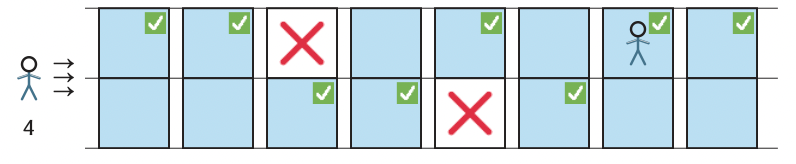The active player, player three, is traversing the bridge and, by observing the outcomes of the hops of the eliminated two players in front of them, has taken only two survival-risking hops to arrive at their current position.

The game proceeds until either all players are eliminated, or all columns of the bridge have been traversed and a subset of the players safely cross the bridge.Continuing with the scenario above, in this outcome (end game), only player four successfully traversed the bridge, without taking any survival-risking hops.

In the Squid Game episode, there are $N=16$ players and the bridge has length $L=18$. Some dramatic elements are neglected in our version but as we’re generalising the number of players and the bridge length, we think this is a fair trade.

Missing here is (i) the time limit to cross the bridge (which, with added pressure, affects the players’ capacity to perfectly remember which panels are tempered), (ii) social dynamics that cause players to act irrationally (eg two players breaking one glass panel in a single manoeuvre, hence not maximising the total survival rate), and (iii) the ability of a player later on in the line to visually discriminate between weak and strong glass panels.

## Probabilistic questions

We raise two questions about the stochastic process in the bridge survival game:

❓ How many of the players do we expect to make it safely across the bridge?
❓ What is the probability that any given player survives?

We can see straight away that the survival of a player in the back of the line is dependent on the outcomes of the players in the front of the line. Players in the back of the line are more likely to survive because they know which panels are strong in the columns hopped on by the players in front of them. Let’s proceed with some mathematical analysis. Given the total number of players $N$ and bridge length $L$, we define:

• ${\color{red}S}_i$ : the event that player $i$ survives
• $\theta$: the number of players that successfully traverse the bridge (a random variable).

Our mathematically refined questions are:

❓ What is the expected value of $\theta$, $\mathbb{E}[ \theta ]$?
❓ What is the probability of event $S_i$, $P(S_i)$, for $i\in\{1,…,N\}$?

## Overview of our approach

A convenient approach to answer our two questions is to first find $P(F_n)$ for $n\in\{0,\dots,N\}$, with

• ${\color{red}F}_n$: the event that exactly $n$ players fall to their death.

Note the events $\{F_0,\dots,F_N\}$ comprise the sample space of outcomes and are mutually exclusive.

The expected number of survivors is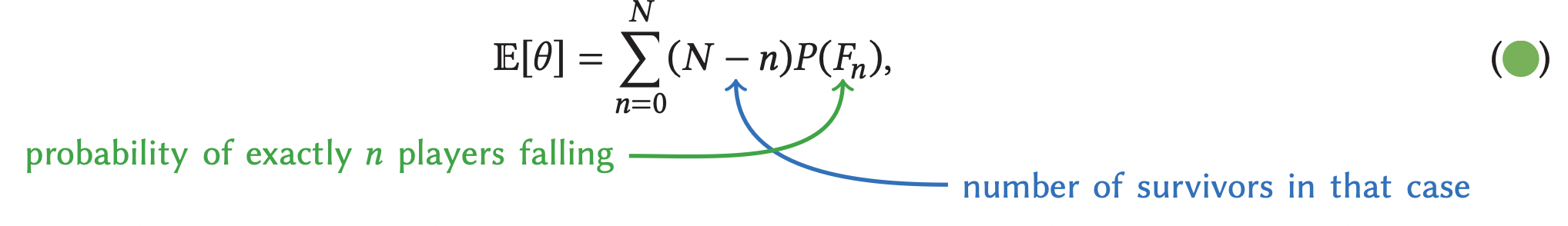since $N-n$ players survive in the event $F_n$.

The probability that player $i$ survives is
$$P(S_i) = \sum_{n=0}^{i-1}P(F_n), \tag{⭐️}$$
since event $S_i$ is the union of the mutually exclusive events $\{F_0,\dots,F_{i-1}\}$, ie $S_i=\bigcup_{n=0}^{i-1}F_n$. In other words, player $i$ survives if it is only players in front of them who fall to their death ($i-1$ or fewer players). For example, this is saying the probability of player four surviving is
\begin{equation*} P(S_4) = P(F_0) + P(F_1) + P(F_2) + P(F_3).  \end{equation*}

What else can we say?

? Each hop by an active player onto a column not yet visited by any player constitutes a trial—specifically a Bernoulli trial—with an outcome of success (survival) with probability $1/2$ and of failure (death) with probability $1/2$. Regardless of the outcome, each trial reveals to the players behind which panel in that column is composed of strong glass. Only after $L$ trials is the entire state of the bridge known, which would reveal to the remaining players—if any—the path for safe bridge traversal.

? Since each Bernoulli trial is independent, the probability of any particular sequence of outcomes of $c$ trials—that is, any particular pattern of broken glass panels on the first $c$ columns of the bridge—is $(1/2)^c$.

We split our analysis of the bridge survival game into two cases:

1. at least as many players as columns, $N \geq L$;
2. more columns than players, $N<L$ (as is the case in the episode!).

Case $N \geq L$ is easier to analyse because all columns of the bridge will certainly be visited, ie $L$ trials will take place. For case $N<L$, in contrast, all players could be eliminated before the final column of the bridge is reached, so we could have $N,N+1,\ldots,L$ trials.

## More players than columns (or equal), $N\geq L$

Suppose the number of players is greater than or equal to the bridge length, $N\geq L$. Then, all $L$ columns of the bridge will be visited. Let’s find the probability of exactly $n$ players falling, $P(F_n)$. If we think about the maximum possible number of deaths, we can make an observation right away: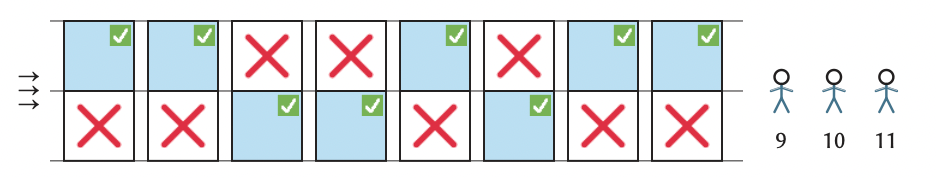Consider the player $n>L$. At most, $L$ players fall to their death. This happens only if, as above, all of the first $L$ players choose to hop onto a weak glass panel in their first hop onto an unvisited column. This outcome would reveal the path for safe traversal over the bridge for the remaining $N-L$ players behind them. Therefore, player $n$ is guaranteed to survive and $P(F_n)=0$ for $n>L$.

So let’s instead think about the player $n\leq L$, and the event that $n$ players fall to their death. The key is to count the number of ways to distribute the $n$ broken glass panels among the $L$ columns of the bridge: \begin{equation*} \binom{L}{n}=\frac{L!}{(L-n)!n!}. \end{equation*} Since each of these distributions of broken glass panels occurs with probability $\left(1/2\right)^L$, $P(F_n)=\binom{L}{n}\left(\frac{1}{2}\right)^L$ for $n\leq L$. Therefore: \begin{equation} P(F_n) = \begin{cases} \binom{L}{n}\left(\frac{1}{2}\right)^L & 0 \leq n \leq L \\ 0 & L < n \leq N. \end{cases} \label{eq:p_en} \tag{?} \end{equation} Another way to arrive here is to consider a binomial distribution: of $2^L$ equally likely outcomes, $\binom{L}{n}$ of them result in $n$ players falling to their death.

Two sanity checks on this result:

1. The probability that zero players fall to their death is $P(F_0)=(1/2)^L$, since then the first player must choose the strong glass panel in each column for each of their $L$ hops to cross the bridge; by similar reasoning, $P(F_L)=(1/2)^L$.
2. Since the events $\{F_0,\dots,F_N\}$ are mutually exclusive and their union comprises the sample space, we must have $\sum_{n=0}^N P(F_n)=1$, which holds since $\sum_{n=0}^L \binom{L}{n}=2^L$ via the binomial theorem.

## More columns than players, $N < L$

Suppose, as in the episode, that the number of players is less than the bridge length, $N < L$. Then, not all $L$ columns of the bridge are necessarily visited, particularly in the event that all $N$ players are eliminated. Unlike the case above, no player is certain to survive, so let’s again find the probability that exactly $n$ players don’t make it, $P(F_n)$.

A useful observation here is the following:

? If exactly $n$ players fall, and $n<N$, then there must be players who made it! In which case, all $L$ columns must be visited and we’re in the same situation as our earlier case. Thus, equation (?) for $P(F_n)$ holds for $n<N$.

So we’re just left with finding $P(F_n)$ for $n=N$. The event $F_N$ (all players eliminated) is the union of all the possible ways player $N$ could have died; ie the mutually exclusive events that player $N$ fell through at column $c=N,\ldots,L$ of the bridge. For each column $c$ at which player $N$ could fall through:

• there are $\binom{c-1}{N-1}$ ways to distribute the broken glass panels of the preceding $N-1$ players onto the previous $c-1$ columns on the bridge;
• each of these distributions of broken glass panels (along with the broken panel at column $c$) occurs with probability $\left(\frac{1}{2}\right)^{c-1}\left(\frac{1}{2}\right) = \left(\frac{1}{2}\right)^c$.

For example, if two players attempt cross a bridge with four columns, the possible outcomes whereby both of them die ($N=2$, $L=4$) are shown below, arranged vertically by $c$, the column player 2 fell through. Each outcome occurs with probability $(1/2)^c$.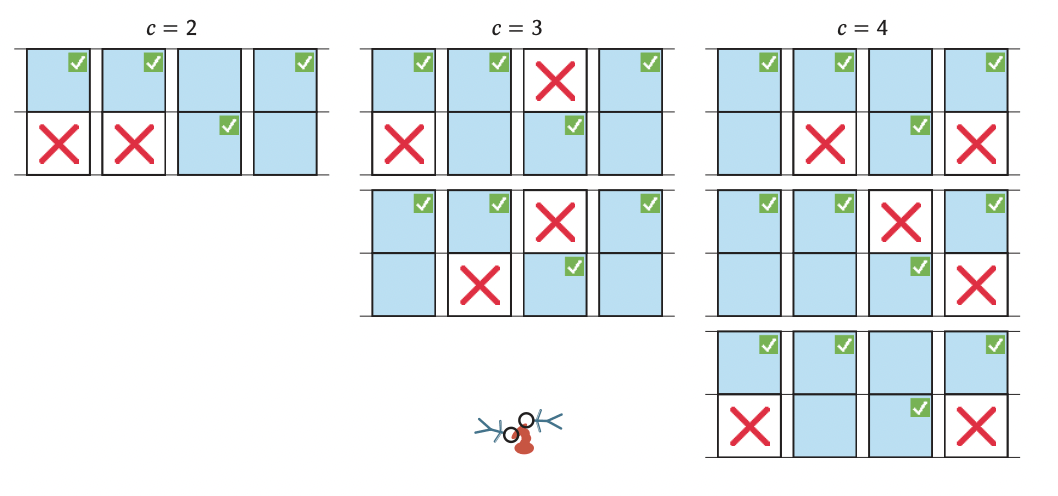To obtain $P(F_N)$, we sum $\binom{c-1}{N-1}\left(\frac{1}{2}\right)^c$ over the possible columns $c\in\{N, \dots,L\}$ at which player $N$ could fall through. Therefore, \begin{equation} P(F_n) = \begin{cases} \binom{L}{n}\left(\frac{1}{2}\right)^L & 0 \leq n < N \\ \sum_{c=N}^L \binom{c-1}{N-1} \left(\frac{1}{2}\right)^c & n=N. \end{cases} \label{eq:p_en2} \tag{☂️} \end{equation} Two sanity checks on this result: (1) It reduces to equation (?) when $N=L$. (2) Again, $\sum_{n=0}^N P(F_n)=1$, a slightly more involved calculation.

## Putting it all together

Equations (?) and (☂️)  give the probability that exactly $n\in\{0,…,N\}$ players do not survive, $P(F_n)$, under cases $N\geq L$ and $N<L$. The expected number of survivors, $\mathbb{E}(\theta)$, and probability that player $i\in\{1,…,N\}$ survives, $P(S_i)$, then follow from equations (?) and (⭐️).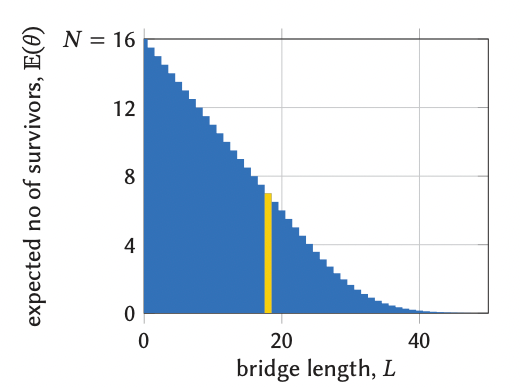In the episode, then, how many of our 16 players do we expect to survive? On the right we plot $\mathbb{E}[\theta]$ as a function of the bridge length, $L$, for $N=16$ total players. As the bridge lengthens, fewer players are expected to survive. For a bridge of length 18, as in the episode, we’d expect seven survivors

What about the actual survival rate of each contestant? Below we show $P(S_i)$ under three different scenarios: (a) $N>L$, (b) $N=L$, (c) $N<L$. We also show $N=16<18=L$ from the episode in (d). We see the players in the back of the line have an advantage since they observe the outcomes of the players in front of them. For our episode scenario in (d), we see our seven expected survivors are the ones with survival probability $P(S_i)>0.5$.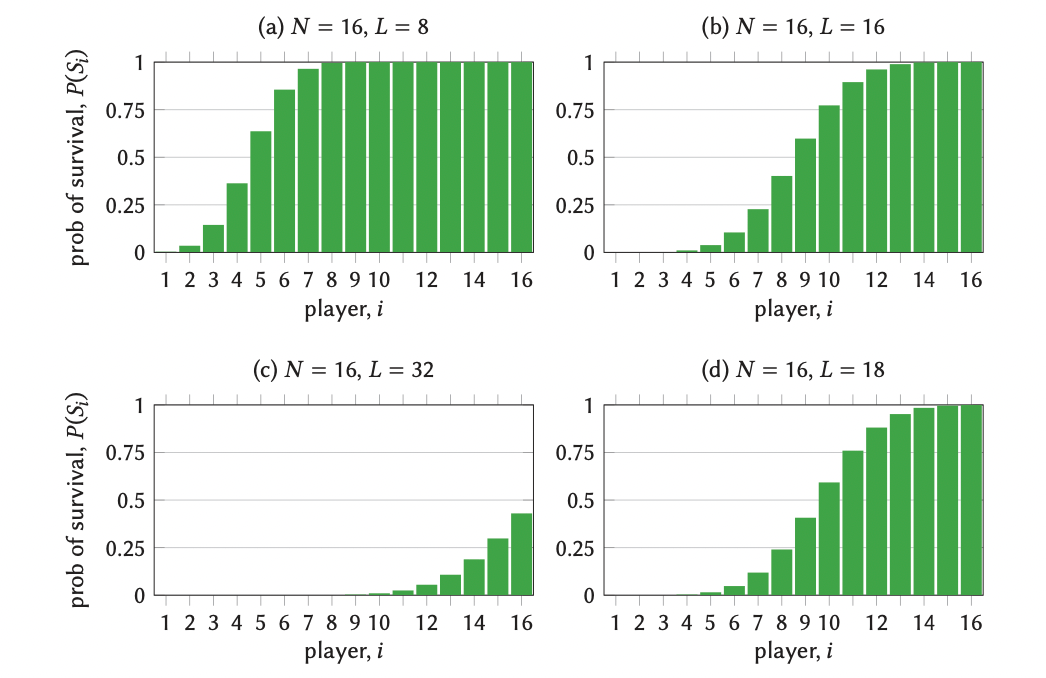But could it have all gone wrong for the organisers? Could all $N=16$ players have been eliminated? For a bridge of length $L=18$ we have that $P(F_{16}) = \sum_{c=16}^{18} \begin{pmatrix} c-1 \\ 16 -1 \end{pmatrix}\left(\frac{1}{2}\right)^c = 0.000656,$ so there was only a 0.07% chance that all players would have died in this round. Good news for the VIPs—99.93% chance of at least one potential Squid Game victor!

## Extensions

There are many possible extensions to the framework that we’ve presented here, which could make for interesting applied probability problems. For instance, inspired by one of the players in Squid Game who worked at a glass factory, incorporating an ability for some player(s) to discern between strong and weak glass panels would impact the probabilities in the Bernoulli trial hops for said players $i \in \{1, \dots, N\}$.

We could also account for imperfect memory of the players of which glass panels of a previously successfully traversed column is the strong one. Maybe this could be done by adding some stochasticity into the framework! Another option, to make the game even harder, could be to add more rows to the bridge (eg three instead of two) and/or vary the distributions of weak versus strong glass panels.

And finally, like in Squid Game, we could include a time limit for the players to cross the bridge and impose a distribution on the time per hop. We suspect this extension would show an optimal position in the line: the first player is unlikely to survive without the advantage of seeing the outcomes of other players in front of them; the last player is unlikely to survive owing to the time limit. The extra-rows extension may be an idea for an enhanced bridge survival game for season 2 of Squid Game!

To help verify our formulas for $P(S_i)$ and $\mathbb{E}[\theta]$, we wrote a Julia code that runs a Monte Carlo simulation of the bridge survival game.Adrian is a doctoral student in chemical engineering at Oregon State University. He researches the application of novel machine learning techniques in predicting properties of nanoporous materials.Nick is a PhD student in physics at Oregon State University researching nanoporous materials and their applications. When off the clock, he likes to hike local trails and do hobby science projects.François-Xavier is a senior researcher at CNRS in France, and professor at PSL University in Paris. He teaches statistical physics, thermodynamics, and modelling of chemical systems.Cory is an assistant professor of chemical engineering at Oregon State University. He enjoys applied mathematics and walks in the forest with his two Samoyeds.